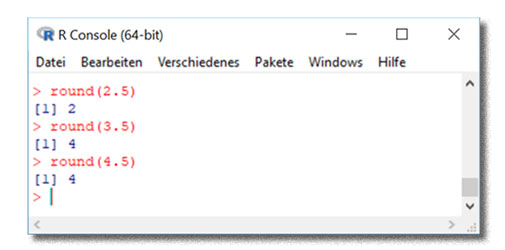R does not round 2.5 to 3
508

# R does not round 2.5 to 3## 18 Sep R does not round 2.5 to 3

In R’s default round() functions, odd numbers ending with .5 are rounded up (1.5 becomes 2), but even numbers are rounded down (2.5 becomes 2, rather than 3). This is compliant with a norm on rounding as outlined in the R documentation, but sometimes inconvenient.

The rounding behavior of R is like this

``````for (i in c(1.5, 2.5, 3.5, 4.5)) print(c(i,round(i,0)))
# original rounded
#  1.5 2.0
#  2.5 2.0
#  3.5 4.0
#  4.5 4.0
``````

You need to round 2.5 to 3 nevertheless? I needed this at some point to break an otherwise infinite loop in my iterative parameter approximation. Here is a solution from Stock Overflow who had it from statistically significant.

``````round2 <- function(x, n) {
posneg = sign(x)
z = abs(x)*10^n
z = z + 0.5
z = trunc(z)
z = z/10^n
z * posneg
}
``````

Where x is the object we want to round, and n is the number of digits just like in the standard rounding function. Rounding behavior looks like this

``````for (i in c(1.5, 2.5, 3.5, 4.5)) print(c(i,round2(i,0)))
# original rounded
#  1.5 2.0
#  2.5 3.0
#  3.5 4.0
#  4.5 5.0
``````